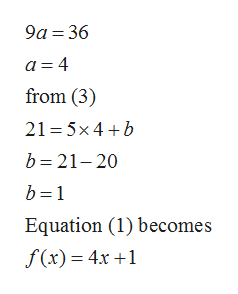# Let f be a linear function for whichf(−4) = −15andf(5) = 21.Determinef(0). f(0) =

Question

Let f be a linear function for which

f(−4) = −15

and

f(5) = 21.

Determine

f(0).

f(0) =
check_circle

Step 1

Given f(x) is a linear function so f(x) is of the form

Step 2

Given f (-4)= -15 and f(5)= 21, putting these values in equation (1)

Step 3

Subtracting equation (2) from (3...help_outlineImage Transcriptionclose9a 36 a 4 from (3) 21 5x4b b 21-20 b 1 Equation (1) becomes f (x) 4x 1 fullscreen

### Want to see the full answer?

See Solution

#### Want to see this answer and more?

Solutions are written by subject experts who are available 24/7. Questions are typically answered within 1 hour.*

See Solution
*Response times may vary by subject and question.
Tagged in

### Other